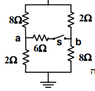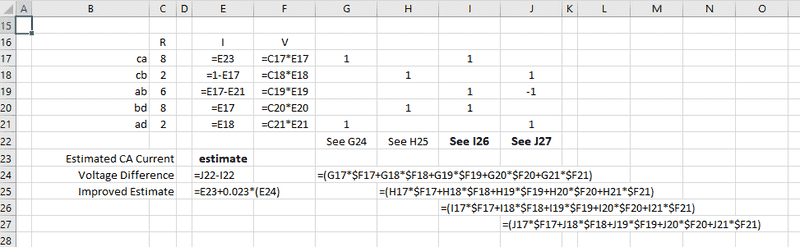# Combined resistance in this circuit

• Eitan Levy
YIn summary, the homework statement is trying to find the combined resistance once the switch is closed. The student used a spread sheet to find the resistance and then used Kirchoff's law to find the voltages.

## Homework Statement

Find the combined resistance once the switch is closed.

V=IR

## The Attempt at a Solution

I tried applying Kirchoff’s law but I got many equations, I am sure there is a more efficient way. I also tried to re-draw the circuit, but wasn't sure how to do so. The answer is 4Ω.

#### Attachments

•‏‏לכידה.PNG
2.6 KB · Views: 395
The resistance is measured from top to bottom.
I do not get exactly 4.

I took node C as the top and D as the bottom, and assumed the current through the network was 1.
Then I set current CA to an estimated value and deduced all other currents (CB, AB, BD, and AD) based on that. Then, given the resistances, I computed all 5 of those voltages. Then I computed the total voltages CA+AB+BD and CB-AB+AD. Then I adjusted my original estimate for CA current to make those two total voltages equal.

That's probably as awkward as any other method. But it gave me an answer.

Last edited:
.Scott said:
The resistance is measured from top to bottom.
I do not get exactly 4.
Perhaps it was rounded. Could you explain how you got it?

Eitan Levy said:
Perhaps it was rounded. Could you explain how you got it?
I just edited post #2 with details.
If you're handy with Excel (or other spread sheet), it is pretty straight forward.

Eitan Levy said:
Could you explain how you got it?Cells F18:21 are copies of F17.
Cells H23:J23 are copies of G23

All 4 values on row 22 should be the total voltage (and therefore impedance) from top (node C) to bottom (node D)

The 0.023 factor in line 25 results from experimentation. I did a few E23 adjustments manually until I saw what the approximate correction factor was. Then I put that into the "Improved Estimate" calculation.

#### Attachments

Eitan Levy said:
I am sure there is a more efficient way.
check out delta / Y transforms (or in your case, Y / delta transforms)

•.Scott